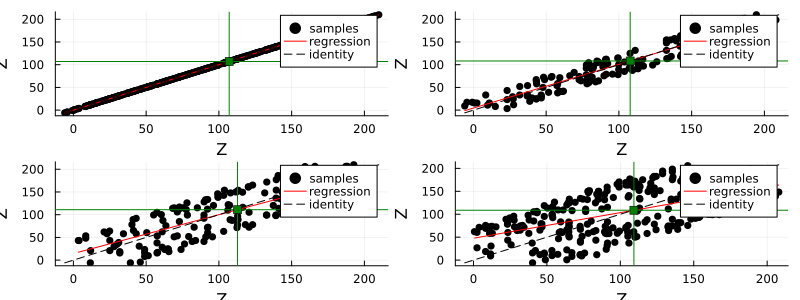# Plotting

Most objects defined in GeoStats.jl can be plotted directly with Plots.jl or Makie.jl. In order to do this, first install GeoStatsPlots.jl (for Plots.jl) or GeoStatsViz.jl (for Makie.jl), which define recipes for each plotting library. Additional plots are listed below that can be useful for geostatistical analysis.

## Built-in

A hscatter plot between two variables var1 and var2 (possibly with var2 = var1) is a simple scatter plot in which the dots represent all ordered pairs of values of var1 and var2 at a given lag h.

using GeoStats
using Plots, GeoStatsPlots

𝒟 = georef((Z=[10sin(i/10) + j for i in 1:100, j in 1:200],))

𝒮 = sample(𝒟, 500)

p1 = hscatter(𝒮, :Z, lag=0)
p2 = hscatter(𝒮, :Z, lag=20)
p3 = hscatter(𝒮, :Z, lag=40)
p4 = hscatter(𝒮, :Z, lag=60)

plot(p1, p2, p3, p4)## PairPlots.jl

The PairPlots.jl package provides the corner plot that can be used with any table, including tables of attributes obtained with the values function.

## StatsPlots.jl

The StatsPlots.jl package provides various statistical plots such as boxplot, dotplot, violin and other plots commonly used in statistical workflows.